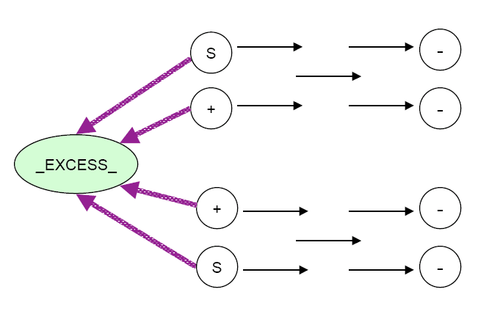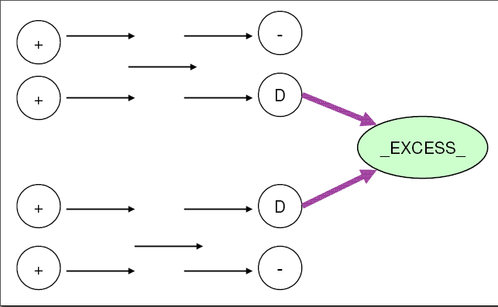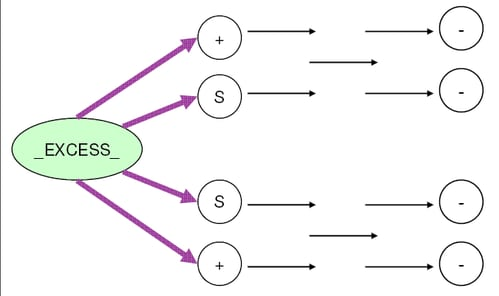### The EXCESS=SUPPLY Option

If you specify the EXCESS=SUPPLY option, then there are three possible scenarios to deal with:

Case 1: No Node with Missing D Demand, THRUNET Not Specified (see Figure 6.33)

Drain the excess supply from all supply nodes.

Figure 6.33: Nodes with Missing S Supply, THRUNET SpecifiedCase 2: Some Nodes with Missing D Demand, THRUNET Not Specified (see Figure 6.34)

Drain the excess supply from nodes that have missing D demand values. If a node has a missing D demand value, then the amount it demands is determined by optimization. For a demand node with negative `supdem` value, that value negated is equal to the sum of flows on all actual arcs directed toward that node.

Figure 6.34: Nodes with Missing D DemandCase 3: THRUNET Specified (see Figure 6.35)

Drain the excess supply from all demand nodes. If a node has a negative `supdem` value, that value negated is the lower bound on the sum of flows on all actual arcs directed toward that node. If a node has a missing D demand value, then the amount it demands is determined by optimization.

Figure 6.35: Nodes with Missing D Demand, THRUNET Specified